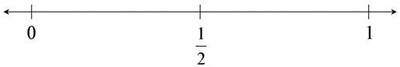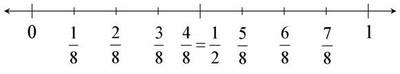Closest to 1/2

Alignments to Content Standards: 3.NF.A.2

Which number is closest to $\frac12$?1. $\frac18$

2. $\frac38$

3. $\frac78$

4. $\frac98$

IM Commentary

How students tackle the problem and the amount of work they show on the number line can provide insight into the sophistication of their thinking. As students partition the interval between 0 and 1 into eighths, they will need to recognize that $\frac12 = \frac48$. Students who systematically plot every point, even $\frac98$, which is larger even than $1$ may still be coming to grips with the relative size of fractions.

The following lists related tasks in order of sophistication:

• Locating Fractions Less than One on the Number Line
• Locating Fractions Greater than One on the Number Line
• Closest to $\frac12$
• Find 1
• Find $\frac23$
• Which is Closer to 1?

Solutions

Solution: Finding $\frac18$ Intervals

(b) is correct.

Many students will mark off eighths in order to see that $\frac38$ is closest to $\frac12$.(So that we can see both perspectives at the same time, note that the middle tickmark has been labelled with both of the equal representations $\frac{4}{8}$ and $\frac{1}{2}$.)

Solution: $\frac38$ is halfway between $\frac14$ and $\frac12$

(b) is correct.

A student who recognizes that $\frac12=\frac48$ and that of the choices, $\frac38$ is closest might just mark
$\frac14=\frac24$ halfway between 0 and $\frac12$ and then mark $\frac38$ halfway between $\frac14$ and $\frac12$.